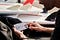Photo by DocuSign on Unsplash

# MuSig: A Secure Method of Merging Public Keys with a Single Signature

The Schnorr signature method supports the merging of public keys to produce a single signature for a transaction [Schnorr aggregate]. Unfortunately, it is not secure and suffers from the cancellation problem [here], but which can be overcome with the MuSig method or the BN Method [here]. In this article we will simplify the method in order to illustrate how it works, and use just two signers (Bob and Alice). The MuSig method is outlined by Greg Maxwell et al in this paper [here]:

To sign a message, Bob takes his private key, a random value (r_i) and a message (msg), and produces a signature: (R, s). Initially, Bob generates a private key of x_1 and a public key of:

X_1=x_1 G

and where G is the base point on the curve (and where X_1 is a point on the curve). Alice will generate her private key (x_2) and a public key of:

X_2=x_2 G

We compute the hash of the merged public keys with:

L=H(X_1||X_2)

Now we can merge their public keys (X) to give:

X=H(L||X_1)X_1+H(L||X_2) X_2

For Bob’s signature, he generates a random value r_1 and computes a point on the curve of:

R1=r_1 G

and Alice computes a point on the curve of:

R2=r_2 G

We can then merge these values to get R with:

R=R1+R2

Bob then computes an s value of:

s_1=r_1+H(X||R||msg) H(L || x1) x1

and where H(X||R||msg) is a hash of the merged public key (X), R and the message. Alice computes her value of:

s_2=r_2+H(X||R||msg) H(L || x1) x2

We can then merge s_1 and s_2 to give:

s=s1+s2

The merged signature of the message is the (R,s). To check we compute the merged signature we compute:

v_1=sG

v_2=R+H(X||R||M)X

If the two values match, the merged signature has been proven. Note that only Bob can produce the correct value of s_1 (as he knows the private key of x_1), and only Alice can produce the correct value of s_2 (as he knows the private key of x_2).

The code used is [here]:

A sample run [here]:

And there you go. If we had a transaction with many signers, we can merge these into a single signature, and then be able to check with the public keys of the entities involved.

## Reference

 Maxwell, G., Poelstra, A., Seurin, Y., & Wuille, P. (2019). Simple schnorr multi-signatures with applications to bitcoin. Designs, Codes and Cryptography, 87(9), 2139–2164 [here].

Professor of Cryptography. Serial innovator. Believer in fairness, justice & freedom. EU Citizen. Auld Reekie native. Old World Breaker. New World Creator.

## More from Prof Bill Buchanan OBE

Professor of Cryptography. Serial innovator. Believer in fairness, justice & freedom. EU Citizen. Auld Reekie native. Old World Breaker. New World Creator.